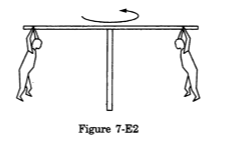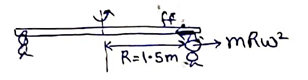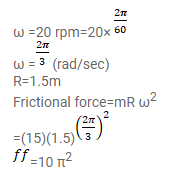# In a children's park a heavy rod is pivoted at the centre

Question:

In a children's park a heavy rod is pivoted at the centre and is made to rotate about the pivot so that the rod always remains horizontal. Two kids hold the rod near the ends and thus rotate with the rod (figure 7-E2). Let the mass of each kid be $15 \mathrm{~kg}$, the distance between the points of the rod where the two kids hold it be $3.0 \mathrm{~m}$ and suppose that the rod rotates at the rate of 20 revolutions per minute. Find the force of friction exerted by the rod on one of the kids.Solution: